# Banker’s Algorithm

Banker’s Algorithm- Operating System - The banker’s algorithm is a resource allocation and deadlock avoidance algorithm that tests for safety

The banker’s algorithm is a resource allocation and deadlock avoidance algorithm that tests for safety by simulating the allocation for predetermined maximum possible amounts of all resources, then makes an “s-state” check to test for possible activities, before deciding whether allocation should be allowed to continue.

Following Data structures are used to implement the Banker’s Algorithm:

Let ‘n’ be the number of processes in the system and ‘m’ be the number of resources types.

Available :

• It is a 1-d array of size ‘m’ indicating the number of available resources of each type.
• Available[ j ] = k means there are ‘k’ instances of resource type Rj

Max :

• It is a 2-d array of size ‘n*m’ that defines the maximum demand of each process in a system.
• Max[ i, j ] = k means process Pi may request at most ‘k’ instances of resource type Rj.

Allocation :

• It is a 2-d array of size ‘n*m’ that defines the number of resources of each type currently allocated to each process.
• Allocation[ i, j ] = k means process Pi is currently allocated ‘k’ instances of resource type Rj

Need :

•  It is a 2-d array of size ‘n*m’ that indicates the remaining resource need of each process.
• Need [ i,  j ] = k means process Pi currently allocated ‘k’ instances of resource type Rj
• Need [ i,  j ] = Max [ i,  j ] – Allocation [ i,  j ]

Allocationi specifies the resources currently allocated to process Pi and Needi specifies the additional resources that process Pi may still request to complete its task.

Banker’s algorithm consist of Safety algorithm and Resource request algorithm

Safety Algorithm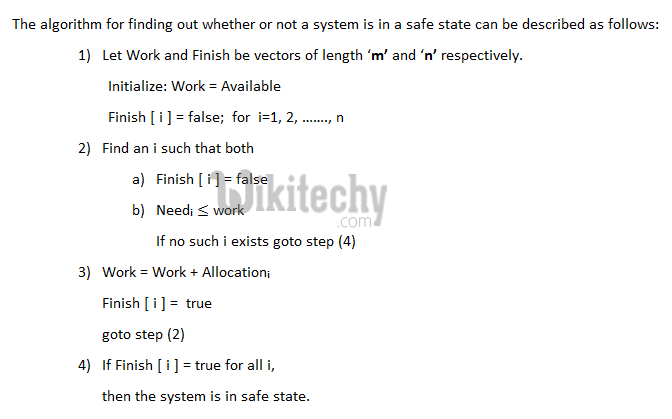Resource-Request Algorithm

Let Requesti be the request array for process Pi. Requesti [j] = k means process Pi wants k instances of resource type Rj. When a request for resources is made by process Pi, the following actions are taken:

READ  C Programming - Longest Increasing SubsequenceExample:

Considering a system with five processes P through P4 and three resources types A, B, C. Resource type A has 10 instances, B has 5 instances and type C has 7 instances. Suppose at time t following snapshot of the system has been taken: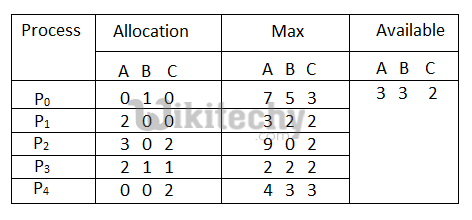Question1. What will be the content of the Need matrix?

Need [i, j] = Max [i, j] – Allocation [i, j]

So, the content of Need Matrix is: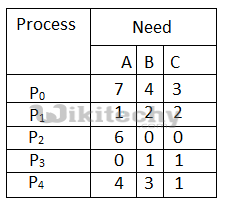Question2.  Is the system in safe state? If Yes, then what is the safe sequence?

Applying the Safety algorithm on the given system,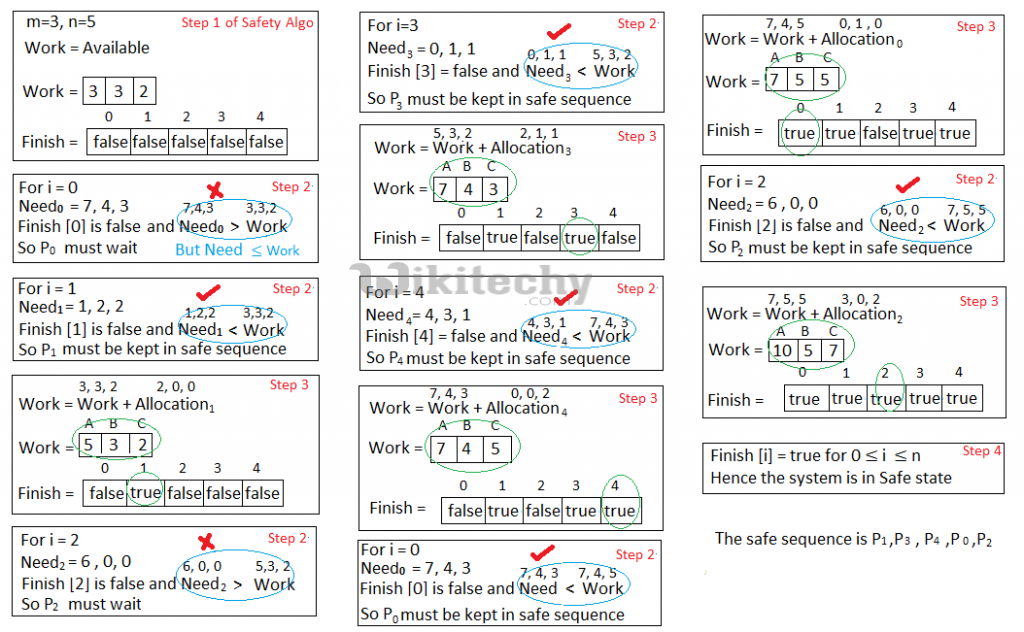Question3. What will happen if process Prequests one additional instance of resource type A and two instances of resource type C?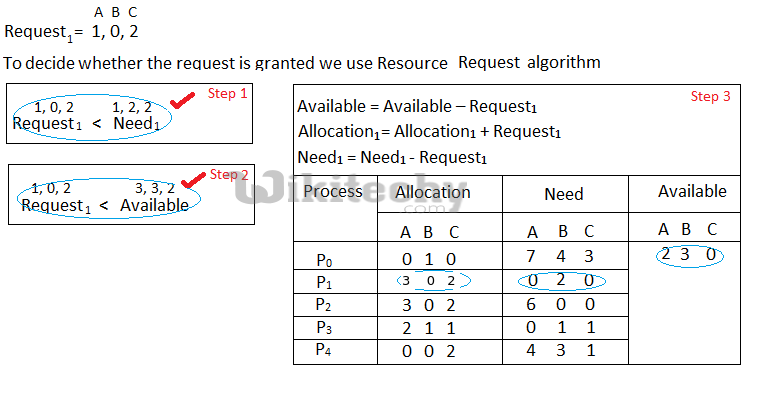We must determine whether this new system state is safe. To do so, we again execute Safety algorithm on the above data structures.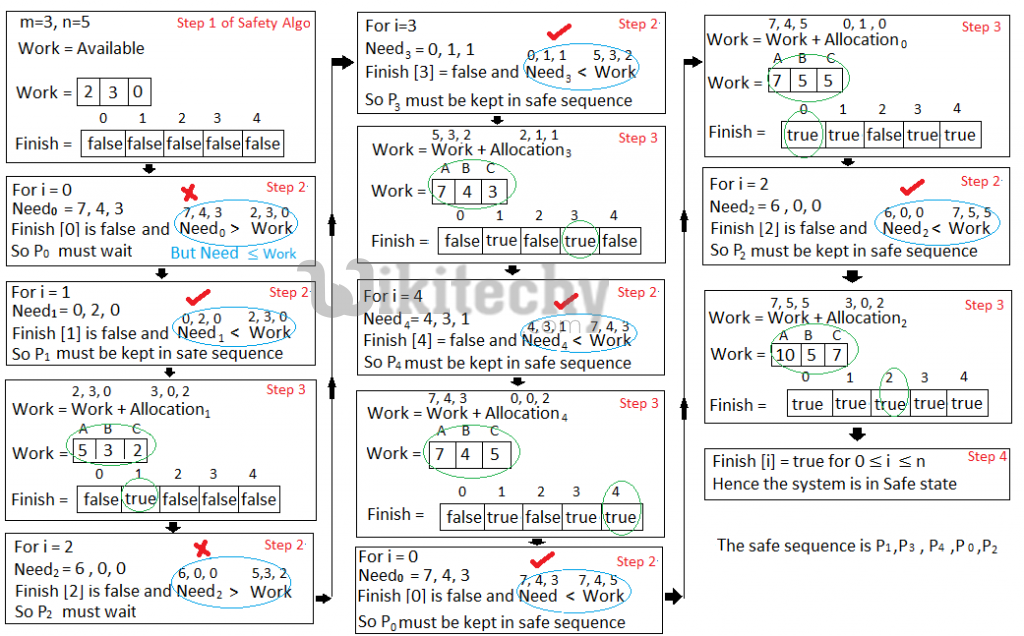Hence the new system state is safe, so we can immediately grant the request for process  P1 .#### Venkatesan Prabu

Wikitechy Founder, Author, International Speaker, and Job Consultant. My role as the CEO of Wikitechy, I help businesses build their next generation digital platforms and help with their product innovation and growth strategy. I'm a frequent speaker at tech conferences and events.

X